: Mathematica Programs : Curious Properties of Reiterated : Loops of the Digit

# 9. Digits Factorial Sum Process.

In this section we are going to study another process that has been proposed by the authors. We define the dfsf function by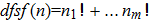, where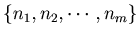is the list of the digits of an integer n . we start with any positive integer n, and repeatedly apply the function dsf, then we can generate a sequence of integers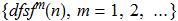.
Example 9.1.   If we apply dfsf function to the number 228702286, then in 13 steps the sequence enters into a loop of three numbers. See Graph 9.1.

Graph 9.1/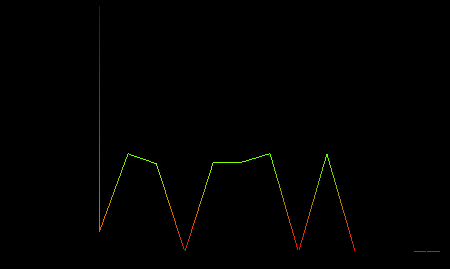If we apply dfsf function to the number 199, then in 33 steps the sequence enters into a loop of three numbers. See Graph 9.2.

Graph 9.2.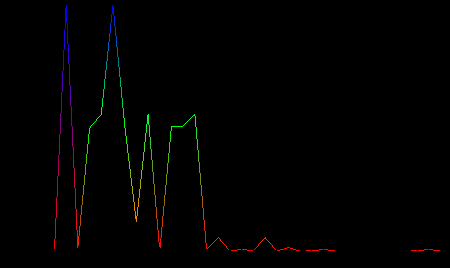Example 9.2.   For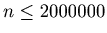the longest steps to enter into a loop is 58, and one of the number that has the longest steps is 1233466.
We are going to present the graph of sequence of numbers in the step to a loop.

Graph 9.3.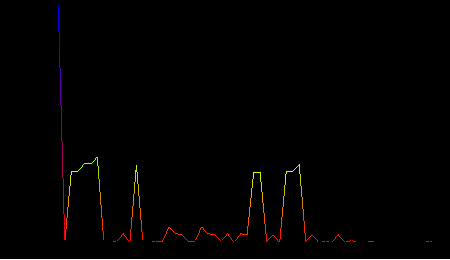: Mathematica Programs : Curious Properties of Reiterated : Loops of the Digit